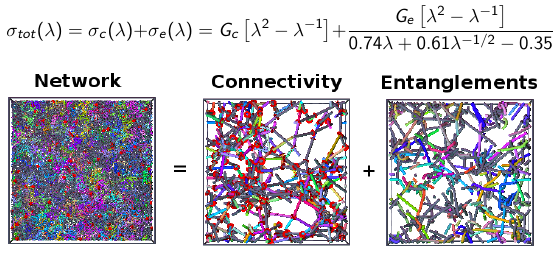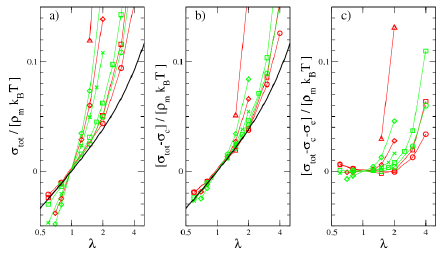Network ageing

Problem: A polymer network is formed by cross-linking a polymer melt. However, the chemical bonds can break due to ageing and deformation. Hence it is of significant interest how the elastic properties are affected by ageing of the network connectivity.

A polymer network has two contributions to the stress-strain relation, one due to the cross-links and the network connectivity, and another due to the topological entanglements. An (completely unvalidated) assumption is that we can simply sum the moduli due to these two effects, this is the idea behind the Rubinstein-Panyukov model:Using molecular dynamics simulations and a variant of our primitive path analysis algorithm, we have measured the total stress as well as the connectivity stress contribution for a large variety of generic bead-spring model polymer networks. For randomly cross- and end-linked networks the inferred entanglement stress contribution is excellent agreement with the predictions of the Rubinstein–Panyukov slip-tube model (Macro-molecules 2002, 35, 6670). For networks undergoing further cross-linking and bond-breaking in the strained state (chemical aging), the observed stress-strain behavior can be described by a generalization of the slip-tube model in the spirit of Tobolsky’s independent network hypothesis. The stress-transfer between independent networks during postcuring appears to obey unexpectedly simple relations.The figure shows a comparison between the total measured stress vs. strain for endlinked and randomly crosslinked networks (red+green symbols) vs. the prediction of the RP model. In the second panel, the connectivity stress contribution has been subtracted, and in the final panel, the residual is shown after also subtracting the entanglement stress contribution.